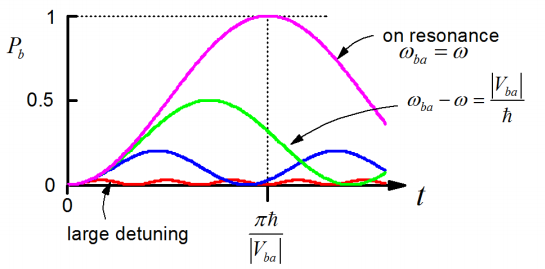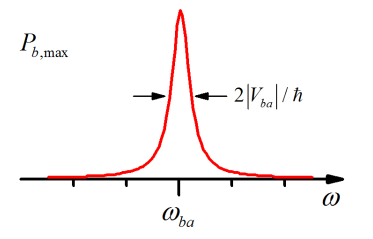# 3.4: Resonant Driving of a Two-Level System

$$\newcommand{\vecs}{\overset { \rightharpoonup} {\mathbf{#1}} }$$ $$\newcommand{\vecd}{\overset{-\!-\!\rightharpoonup}{\vphantom{a}\smash {#1}}}$$$$\newcommand{\id}{\mathrm{id}}$$ $$\newcommand{\Span}{\mathrm{span}}$$ $$\newcommand{\kernel}{\mathrm{null}\,}$$ $$\newcommand{\range}{\mathrm{range}\,}$$ $$\newcommand{\RealPart}{\mathrm{Re}}$$ $$\newcommand{\ImaginaryPart}{\mathrm{Im}}$$ $$\newcommand{\Argument}{\mathrm{Arg}}$$ $$\newcommand{\norm}{\| #1 \|}$$ $$\newcommand{\inner}{\langle #1, #2 \rangle}$$ $$\newcommand{\Span}{\mathrm{span}}$$ $$\newcommand{\id}{\mathrm{id}}$$ $$\newcommand{\Span}{\mathrm{span}}$$ $$\newcommand{\kernel}{\mathrm{null}\,}$$ $$\newcommand{\range}{\mathrm{range}\,}$$ $$\newcommand{\RealPart}{\mathrm{Re}}$$ $$\newcommand{\ImaginaryPart}{\mathrm{Im}}$$ $$\newcommand{\Argument}{\mathrm{Arg}}$$ $$\newcommand{\norm}{\| #1 \|}$$ $$\newcommand{\inner}{\langle #1, #2 \rangle}$$ $$\newcommand{\Span}{\mathrm{span}}$$

Let’s describe what happens when you drive a two-level system with an oscillating potential.

$V (t) = V \cos \omega t \label{2.61}$

$V _ {k \ell} (t) = V _ {k \ell} \cos \omega t \label{2.62}$

Note, this is the form you would expect for an electromagnetic field interacting with charged particles, i.e. dipole transitions. In a simple sense, the electric field is $$\overline {E} (t) = \overline {E} _ {0} \cos \omega t$$ and the interaction potential can be written as $$V = - \overline {E} \cdot \overline {\mu},$$ where $$\overline {\mu}$$ represents the dipole operator.

We will look at the form of this interaction a bit more carefully later. We now couple two states $$| a \rangle$$ and $$| b \rangle$$ with the oscillating field. Here the energy of the states is ordered so that $$\varepsilon _ {b} > \varepsilon _ {a}$$. Let’s ask if the system starts in $$| a \rangle$$ what is the probability of finding it in $$| a \rangle$$ at time $$t$$ ?The system of differential equations that describe this problem is:

\begin{align} i \hbar \frac {\partial} {\partial t} b _ {k} (t) & = \sum _ {n = a , b} b _ {n} (t) V _ {k n} (t) e^{- i \omega _ {n k} t} \\[4pt] & = \sum _ {n = a , b} b _ {n} (t) V _ {k n} e^{- i \omega _ {n k} t} \cdot \frac {1} {2} \left( e^{- i \omega t} + e^{i \omega t} \right) \label{2.63} \end{align}

Where $$\cos \omega t$$ in written its complex form. Writing this explicitly

$i \hbar \dot {b} _ {b} = \frac {1} {2} b _ {a} V _ {b a} \left[ e^{i \left( \omega _ {b a} - \omega \right) t} + e^{i \left( \omega _ {b a} + \omega \right) t} \right] + \frac {1} {2} b _ {b} V _ {b b} \left[ e^{i \omega t} + e^{- i \omega t} \right]$

$i \hbar \dot {b} _ {a} = \frac {1} {2} b _ {a} V _ {a a} \left[ e^{i \omega t} + e^{- i \omega t} \right] + \frac {1} {2} b _ {b} V _ {a b} \left[ e^{i \left( \omega _ {a b} - \omega \right) t} + e^{i \left( \omega _ {a b} + \omega \right) t} \right]$

or alterntively changing the last term:

$i \hbar \dot {b} _ {a} = \frac {1} {2} b _ {a} V _ {a a} \left[ e^{i \omega t} + e^{- i \omega t} \right] + \frac {1} {2} b _ {b} V _ {a b} \left[ e^{- i \left( \omega _ {b a} + \omega \right) t} + e^{- i \left( \omega _ {b a} - \omega \right) t} \right]$

Here the expressions have been written in terms of the frequency $$\omega_{ba}$$. Two of these terms are dropped, since (for our case) the diagonal matrix elements $$V_{ii} =0$$. We also make the secular approximation (or rotating wave approximation) in which the nonresonant terms are dropped. When $$\omega _ {b a} \approx \omega$$, terms like $$e^{\pm i \omega t}$$ or $$e^{i \left( \omega _ {b a} + \omega \right) t}$$ oscillate very rapidly (relative to $$\left| V _ {b a} \right|^{- 1}$$) and so do not contribute much to change of $$c_n$$. (Remember, we take the frequencies $$\omega_{b a}$$ and $$\omega$$ to be positive). So now we have:

$\dot {b} _ {b} = \frac {- i} {2 \hbar} b _ {a} V _ {b a} e^{i \left( \omega _ {b a} - \omega \right) t} \label{2.66}$

$\dot {b} _ {a} = \frac {- i} {2 \hbar} b _ {b} V _ {a b} e^{- i \left( \omega _ {b a} - \omega \right) t} \label{2.67}$

Note that the coefficients are oscillating at the same frequency but phase shifted to one another. Now if we differentiate Equation \ref{2.66}:

$\ddot {b} _ {b} = \frac {- i} {2 \hbar} \left[ \dot {b} _ {a} V _ {b a} e^{i \left( \omega _ {b a} - \omega \right) t} + i \left( \omega _ {b a} - \omega \right) b _ {a} V _ {b a} e^{i \left( \omega _ {b a} - \omega \right) t} \right] \label{2.68}$

Rewrite Equation \ref{2.66}:

$b _ {a} = \frac {2 i \hbar} {V _ {b a}} \dot {b} _ {b} e^{- i \left( \omega _ {b a} - \omega \right) t} \label{2.69}$

and substitute Equations \ref{2.69} and \ref{2.67} into Equation \ref{2.68}, we get linear second order equation for $$b_b$$.

$\ddot {b} _ {b} - i \left( \omega _ {b a} - \omega \right) \dot {b} _ {b} + \frac {\left| V _ {b a} \right|^{2}} {4 \hbar^{2}} b _ {b} = 0 \label{2.70}$

This is just the second order differential equation for a damped harmonic oscillator:

$a \ddot {x} + b \dot {x} + c x = 0 \label{2.71}$

$x = e^{- ( b / 2 a ) t} ( A \cos \mu t + B \sin \mu t ) \label{2.72}$

with

$\mu = \frac {1} {2 a} \sqrt {4 a c - b^{2}}$

With a little more manipulation, and remembering the initial conditions $$b_j(0)=0$$ and $$b_{\ell} (0) =1$$, we find

$P _ {b} (t) = \left| b _ {b} (t) \right|^{2} = \frac {\left| V _ {b a} \right|^{2}} {\left| V _ {b a} \right|^{2} + \hbar^{2} \left( \omega _ {b a} - \omega \right)^{2}} \sin^{2} \Omega _ {R} t \label{2.73}$

Where the Rabi Frequency

$\Omega _ {R} = \frac {1} {2 \hbar} \sqrt {\left| V _ {b a} \right|^{2} + \hbar^{2} \left( \omega _ {b a} - \omega \right)^{2}} \label{2.74}$

Also,

$P _ {a} = 1 - P _ {b} \label{2.75}$

The amplitude oscillates back and forth between the two states at a frequency dictated by the coupling between them. [Note a result we will return to later: electric fields couple quantum states, creating coherences!]

An important observation is the importance of resonance between the driving potential and the energy splitting between states. To get transfer of probability density you need the driving field to be at the same frequency as the energy splitting. On resonance, you always drive probability amplitude entirely from one state to another.The efficiency of driving between $$| a \rangle$$ and $$|b \rangle$$ states drops off with detuning. Here plotting the maximum value of $$P_b$$ as a function of frequency:3.4: Resonant Driving of a Two-Level System is shared under a CC BY-NC-SA 4.0 license and was authored, remixed, and/or curated by Andrei Tokmakoff via source content that was edited to conform to the style and standards of the LibreTexts platform; a detailed edit history is available upon request.